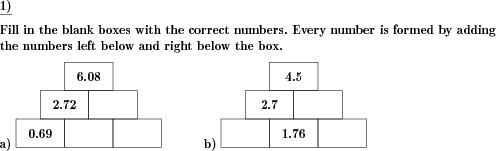Custom math worksheets at your fingertips# Details for problem "Number wall sums of decimal numbers"

Quickname: 5950

Elementary School, Primary School, Junior High School, Middle School, High School.

## Summary

In a number wall, blanks have to be filled in with decimal numbers.

## Example## Description

This is a classic number wall problem, where decimal numbers are summed up ascending through the wall.

The number for each brick is formed by adding the numbers in the brick left below and right below the brick. Some bricks are left blank - these have to be filled in with the correct number. Depending on whether both values below an empty box are given, this may be an addition or a subtraction problem.

The number range and the number of decimal places for the numbers occuring in the number wall can be specified. The wall height is selectable.

Download free printable worksheets for this math problem here. The worksheet contains the problems only, the solution sheet includes the answers. Just click on the respective link.

•Worksheet 1Solution sheet with answers
•Worksheet 2Solution sheet with answers
•Worksheet 3Solution sheet with answers

If you can not see the solution sheets for download, they may be filtered out by an ad blocker that you may have installed. If this is the case, please allow ads for this page and reload the page. The solution sheets will then reappear.

• Do these sample worksheets do not really fit?
• Do you need more math worksheets, with a different level of difficulty?
• Would you like to combine different problems on a worksheet and adjust them to your needs?
• As a teacher, you can put together your own worksheets using the automatically generated math problems provided.
With a free initial credit, you can start creating your own math worksheets in a few minutes.

You can try it for free! Register here, to create custom worksheets now!

## Customization options for this problem

Parameter
Possible values
Number of problems
1, 2, 3, 4, 5, 6, 7, 8, 9, 10
Number range
1, 10, 20, 50, 100
Height of wall
three, four
Number of decimal places
1, 2, 3, 4

## Similar problems

Remark
Description
Number wall with addition of whole numbers
In the number wall missing sums have to be added.Deutsche Version dieser Aufgabe
These informational pages with samples describe math problems that can be combined on custom math worksheets with solutions for home and K-12 school use.
Deutsche Seiten
×